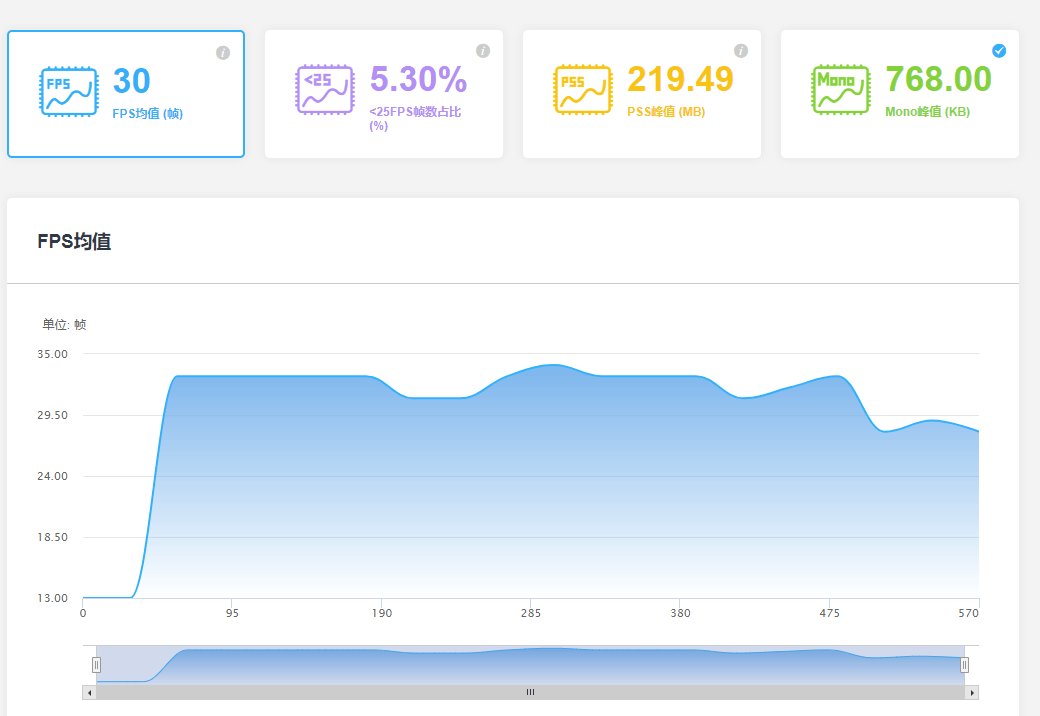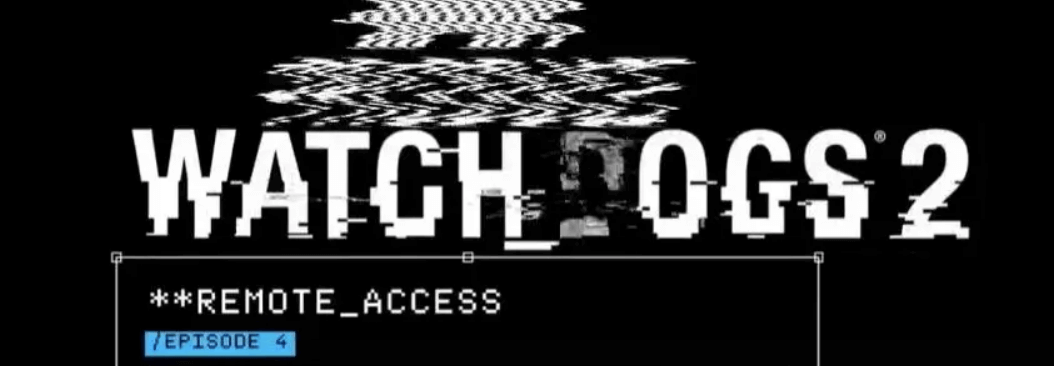【博物纳新】是UWA旨在为开发者推荐新颖、易用、有趣的开源项目，帮助大家在项目研发之余发现世界上的热门项目、前沿技术或者令人惊叹的视觉效果，并探索将其应用到自己项目的可行性。很多时候，我们并不知道自己想要什么，直到某一天我们遇到了它。

## 导读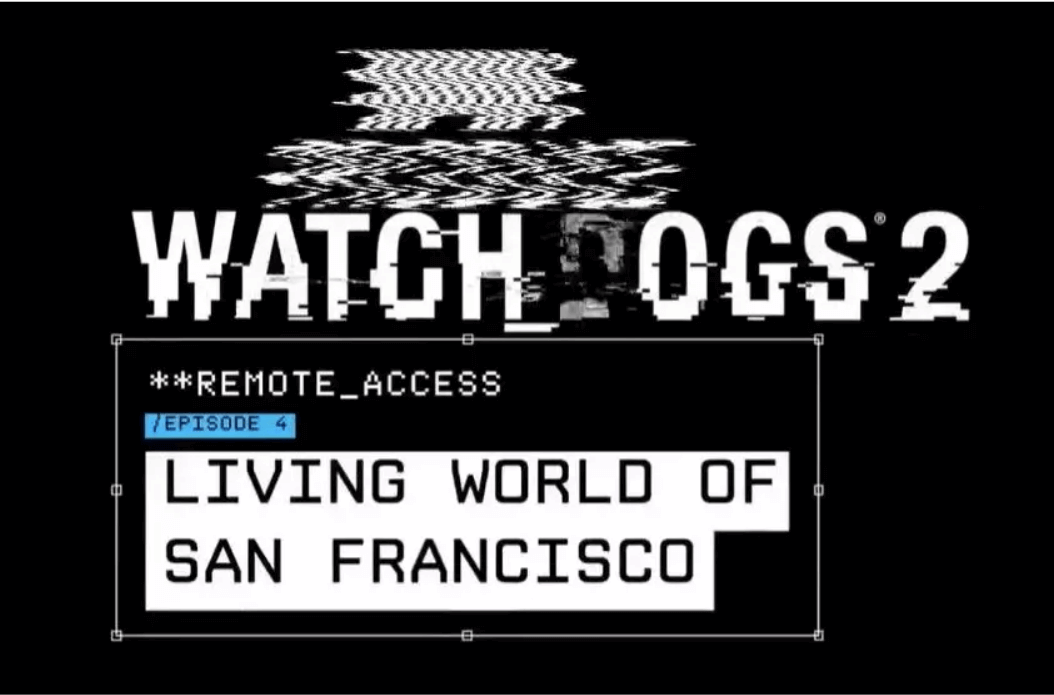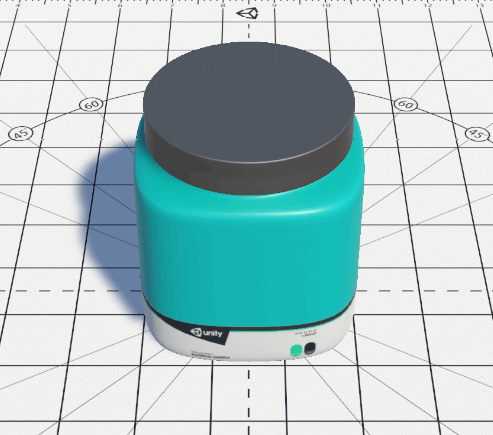（无特效时场景）

## Analog Glitch

1、Scan Line Jitter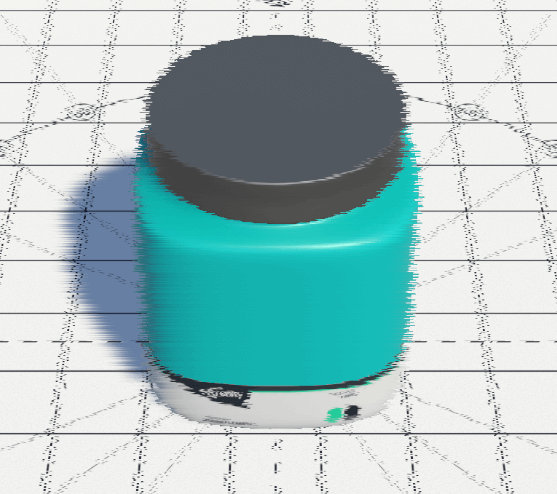（_scanLineJitter设定为0.5时 效果图）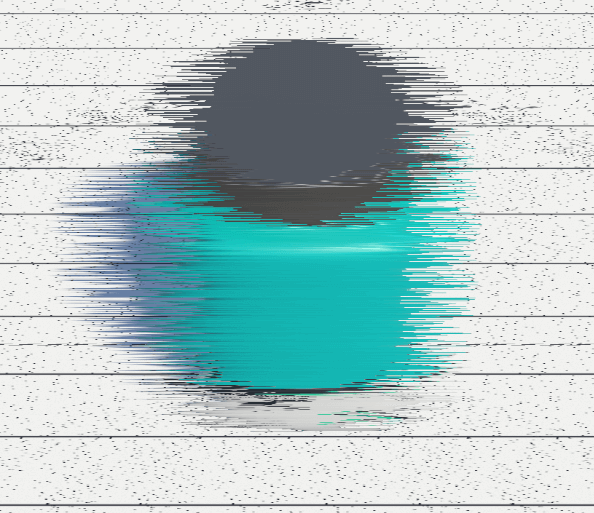（_scanLineJitter设定为1时 效果图）

``````//AnalogGlitch.cs中设定变量用于控制偏移量
[SerializeField, Range(0, 1)]
float _scanLineJitter = 0;

//计算具体偏移量
float jitter = nrand(v, _Time.x) * 2 - 1;
jitter *= step(_ScanLineJitter.y, abs(jitter)) * _ScanLineJitter.x;

float nrand(float x, float y){
return frac(sin(dot(float2(x, y), float2(12.9898, 78.233))) * 43758.5453);
}
//根据偏移量进行采样
half4 src1 = tex2D(_MainTex, frac(float2(u + jitter, v)));
``````

2、Horizontal Shake

``````//AnalogGlitch.cs中设定变量用于控制偏移量
_material.SetFloat("_HorizontalShake", _horizontalShake * 0.2f);
//计算具体偏移
float shake = (nrand(_Time.x, 2) - 0.5) * _HorizontalShake;

float nrand(float x, float y){
return frac(sin(dot(float2(x, y), float2(12.9898, 78.233))) * 43758.5453);
//根据偏移量进行采样
half4 src1 = tex2D(_MainTex, frac(float2(u + shake, v)));
``````

3、Color Drift（效果图）

``````//AnalogGlitch.cs中设定变量用于控制偏移量
var cd = new Vector2(_colorDrift * 0.04f, Time.time * 606.11f);
_material.SetVector("_ColorDrift", cd);
//计算具体偏移
float drift = sin(jump + _ColorDrift.y) * _ColorDrift.x;

//采样原图&根据偏移量进行采样
half4 src1 = tex2D(_MainTex, frac(float2(u , v)));
half4 src2 = tex2D(_MainTex, frac(float2(u + drift, v)));

//将上述的采样结果进行混合
return half4(src1.r, src2.g, src1.b, 1);
``````

4、Vertical Jump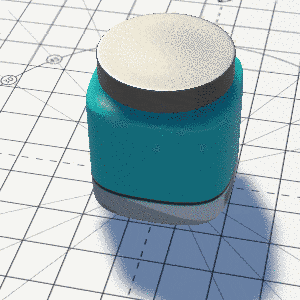（效果图）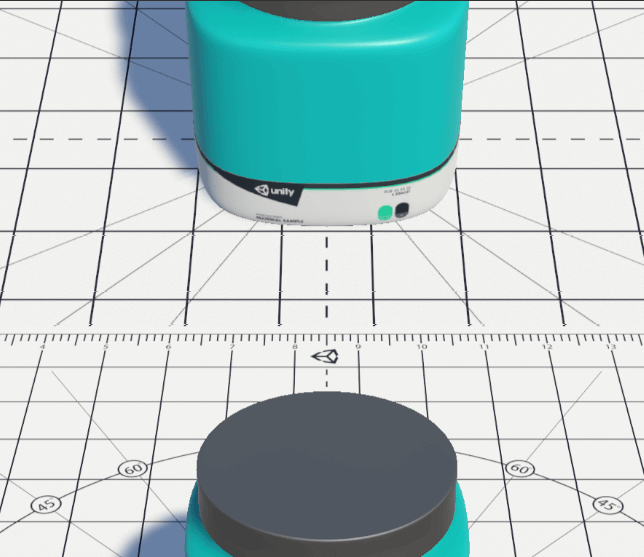（效果图）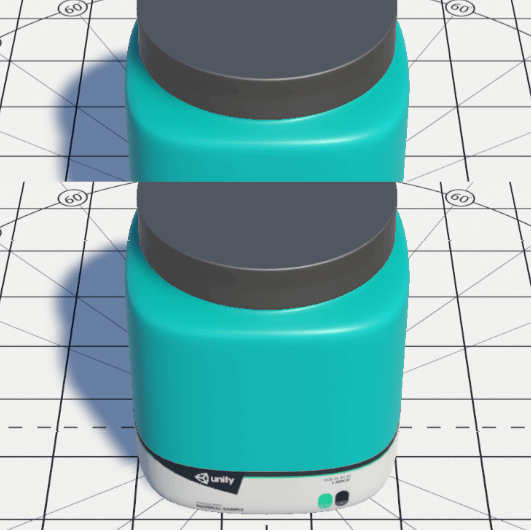（进行插值后效果图）

``````//AnalogGlitch.cs中设定变量用于控制偏移量
_verticalJumpTime += Time.deltaTime * _verticalJump * 11.3f;
var vj = new Vector2(_verticalJump, _verticalJumpTime);
_material.SetVector("_VerticalJump", vj);

//进行插值
float jump = lerp(v, frac(v + _VerticalJump.y), _VerticalJump.x);
//根据偏移量进行采样
half4 src1 = tex2D(_MainTex, frac(float2(u, jump)));
``````

``````half4 src1 = tex2D(_MainTex, frac(float2(u + jitter + shake, jump)));
half4 src2 = tex2D(_MainTex, frac(float2(u + jitter + shake + drift, jump)));
return half4(src1.r, src2.g, src1.b, 1);
``````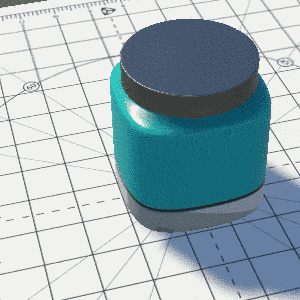(效果图)

## Digital Glitch: Block Damage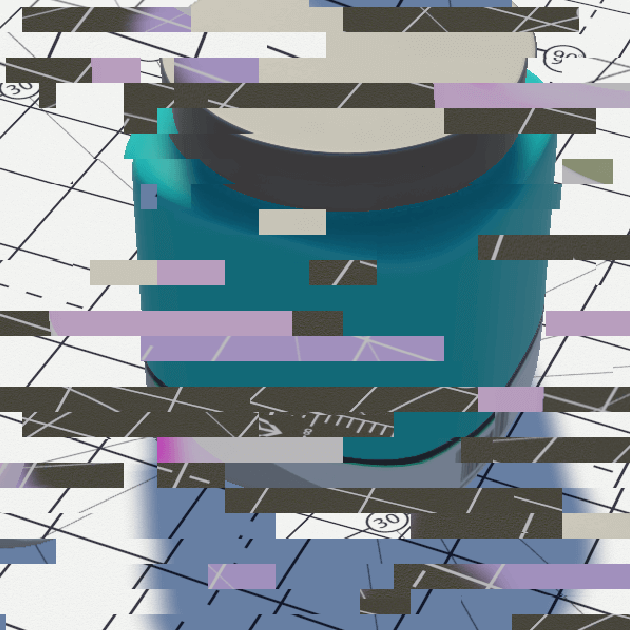（效果图）

``````void UpdateNoiseTexture(){
var color = RandomColor();
for (var y = 0; y < _noiseTexture.height; y++){
for (var x = 0; x < _noiseTexture.width; x++){
if (Random.value > 0.89f)
color = RandomColor();
_noiseTexture.SetPixel(x, y, color);
}
}
_noiseTexture.Apply();
}
``````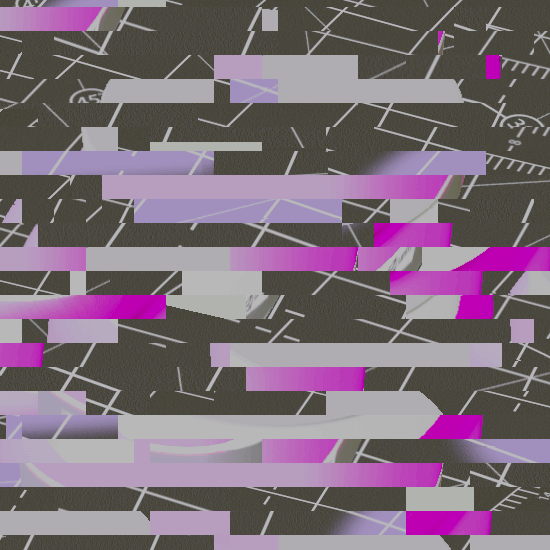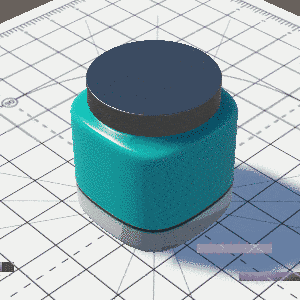## 性能测评